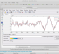## Python (numpy) to Matlab/Octave conversion problemStarted by 1 year ago4 replieslatest reply 1 year ago152 views

I'm new to python language. I can't figure out what happens here in code:

def evaluate(self, x):
p1 = 1
p2 = np.zeros((1, self.r), dtype=self.dtype)
p2 += self.coeff[0, np.newaxis, :]
for k in range(1, self.n):
v = x - self.xcoord[k-1]
p1 = v*p1
p2 += p1[:, np.newaxis] * self.coeff[k]

return p2

where,
n = array size of xcoord
r = self.ycoord.shape
coeff = np.zeros((self.n+1, self.r), dtype=self.dtype)

How these

self.coeff[0, np.newaxis, :]

and

p1[:, np.newaxis]

can be converted to Matlab/Octave language ?

p1 is defined as variable but, then later it is used as an array type variable. coeff is an array type variable ... but, what is done in command

self.coeff[0, np.newaxis, :]

?

[ - ]In NumPy,

np.newaxis

is used to add 'dimension' to the vector or matrix.

The * is used for one-to-one multiplication of elements (not matrix product). I guess 'np.newaxis' is used to have matching matrix dimensions

[ - ]Yes, it looks like np.newaxis does not (in these cases) change the dimensions so, the function can be converted to Matlab/Octave language as like:

 % evaluation  p1 = 1;  p2 = zeros(1, 1);  p2 += coeff(1);  for k = 1:n    v = x - xcoord(k);    p1 = v*p1;    p2 += p1 * coeff(k+1);  endfor
[ - ]Hi,

p2 += p1[:, np.newaxis] * self.coeff[k]

means

p2 = p2 + p1[:, np.newaxis] * self.coeff[k]
[ - ]